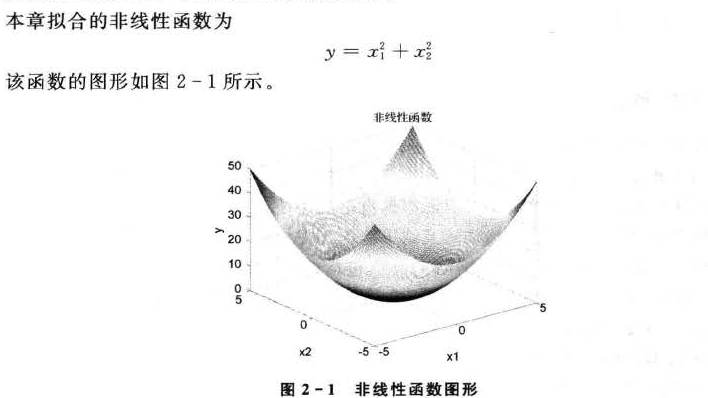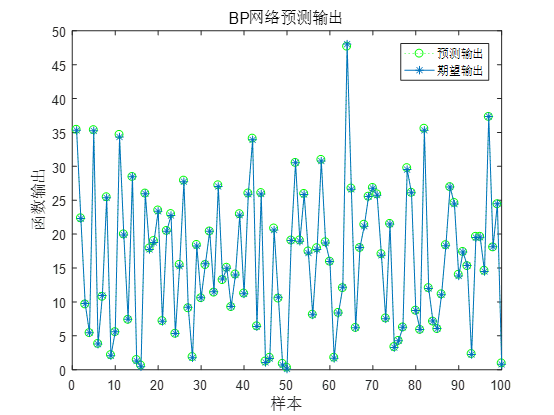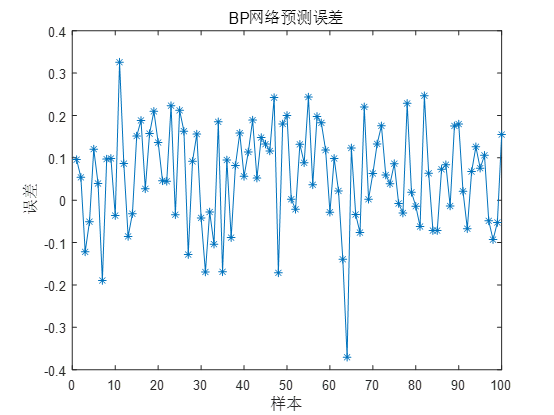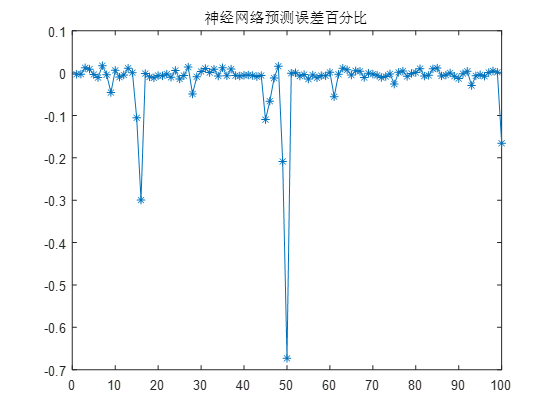• 前两天练题的时候要用到多元非线性拟合，但是在网上找到的大多都是一些求问的帖子，真正有用的回答却不多，这里汇总一下与大家分享。 首先导入数据（参数和结果） 确定自己要拟合函数（自己画个散点图预估一下，...
Process
前两天练题的时候要用到多元非线性拟合，但是在网上找到的大多都是一些求问的帖子，真正有用的回答却不多，这里汇总一下与大家分享。
首先导入数据（参数和结果）确定自己要拟合的函数（自己画个散点图预估一下，或者是使用SPSS的曲线估算，但是没自己画图看的舒服）确定要拟合的系数的预估值（全部写零也行，但是你得有个大概想法，比如你图像是幂次为负的幂函数，那么你就写个负值，比如-1）套用nlinfit函数，得出的bate矩阵就是你要拟合的系数矩阵。covB回归系数的协方差 mse均方误差 beta参数最优值 r各点处的拟合残差 J雅各比矩阵的数值 ErrorModelInfo错误模型信息
Code
clear all;
s=a(:, 6);%y值(结果矩阵)
myfunc=inline('beta(1).*x(:,1).^(beta(2))+beta(3).*x(:,2).^(beta(4))+beta(5).*x(:,3).^(beta(6))+beta(7).*x(:,4).^(beta(8))+beta(9)','beta','x');%内联函数（beta是函数自带的代表待确定的系数）
beta0 = [1, -2 ,1, -2 ,1, -2 ,1, -2 ,60];%待定系数的预估值
[beta,R,J,CovB,MSE,ErrorModelInfo]= nlinfit(b, s, myfunc,beta0);
beta

展开全文• 使用非线性函数拟合 使用线性函数+非线性函数（激活函数去拟合） 为了和神经网络相统一，用线性函数+激活函数去拟合非线性函数 3.搭建网络 分两路进行全连接层，然后将输出拼接，再经过三个全连接层 4.代码实现 ...


文章目录
1. 线性函数2. 非线性函数3.搭建网络4.代码实现5.拟合效果参考

1. 线性函数
线性函数用于拟合线性的曲线
2. 非线性函数
非线性函数怎么拟合呢？
使用非线性函数拟合使用线性函数+非线性函数（激活函数去拟合） 为了和神经网络相统一，用线性函数+激活函数去拟合非线性函数
3.搭建网络分两路进行全连接层，然后将输出拼接，再经过三个全连接层
4.代码实现
使用Tensorboard查看数据
from numpy.core.function_base import linspace
import torch
import numpy as np
import matplotlib.pyplot as plt
from torch import nn, optim
import torch.nn.functional as F
from matplotlib.animation import FuncAnimation
from torch.utils.tensorboard import SummaryWriter

xx = linspace(0, 2, 3000)
class Data(Dataset):
def __init__(self):
super(Data, self).__init__()
self.x = linspace(0, 3.14, 3000)
self.y = []
for i in self.x:
'''
# 阶梯函数
if i <=1:
self.y.append(0)
else:
self.y.append(1)
# 方波函数
if i <=0.5 or i >= 1.5:
self.y.append(0)
else:
self.y.append(1)
# 正弦函数
self.y = np.sin(self.x*2)
'''
self.y = np.sin(self.x*2)

def __len__(self):
return len(self.x)
def __getitem__(self, index):
x = self.x[index]
y = self.y[index]
x = torch.tensor(x).unsqueeze(dim=0).float()
y = torch.tensor(y).unsqueeze(dim=0).float()
return x, y

train = Data()

class Net(nn.Module):
def __init__(self):
super(Net, self).__init__()
self.fc1 = nn.Linear(1, 10)
self.fc2 = nn.Linear(1, 10)
self.fc3 = nn.Linear(20, 100)
self.fc4 = nn.Linear(100, 50)
self.fc5 = nn.Linear(50, 1)
def forward(self, x):
x1 = F.relu(self.fc1(x))
x2 = F.relu(self.fc2(x))
x3 = torch.cat((x1, x2), dim=1)
x = self.fc3(x3)
x = F.relu(self.fc4(x))
x = self.fc5(x)
return x

net = Net()
loss_fn = nn.MSELoss()

for epoch in range(10000):
for x, y in train_data:
y_pred = net(x)
loss = loss_fn(y_pred, y)
print(f"\r第{epoch}个epoch的损失为{loss.item()}", end="")
loss.backward()
optimizer.step()
if epoch%50 == 0:
fig = plt.figure()
subfig1.plot(x.detach().numpy(), y_pred.detach().numpy())
subfig1.plot(x.detach().numpy(), y.detach().numpy())
writer = SummaryWriter()
# plt.show()

使用Matplotlib查看变化动画
from numpy.core.function_base import linspace
import torch
import numpy as np
import matplotlib.pyplot as plt
from torch import nn, optim
import torch.nn.functional as F
from matplotlib.animation import FuncAnimation
from torch.utils.tensorboard import SummaryWriter
import matplotlib.pyplot as plt
from matplotlib.animation import FuncAnimation

xx = linspace(0, 2, 3000)
class Data(Dataset):
def __init__(self):
super(Data, self).__init__()
self.id = 1
self.x = linspace(0, 3.14, 3000)
self.y = []
for i in self.x:
if self.id ==0 :
# 阶梯函数
if i <=1:
self.y.append(0)
else:
self.y.append(1)
elif self.id == 1:
# 方波函数
if i <=0.5 or i >= 1.5:
self.y.append(0)
else:
self.y.append(1)
elif self.id == 2:
# 正弦函数
self.y = np.sin(self.x*2)
self.y = np.sin(self.x*2)

def __len__(self):
return len(self.x)
def __getitem__(self, index):
x = self.x[index]
y = self.y[index]
x = torch.tensor(x).unsqueeze(dim=0).float()
y = torch.tensor(y).unsqueeze(dim=0).float()
return x, y

train = Data()

class Net(nn.Module):
def __init__(self):
super(Net, self).__init__()
self.fc1 = nn.Linear(1, 10)
self.fc2 = nn.Linear(1, 10)
self.fc3 = nn.Linear(20, 100)
self.fc4 = nn.Linear(100, 50)
self.fc5 = nn.Linear(50, 1)
def forward(self, x):
x1 = F.relu(self.fc1(x))
x2 = F.relu(self.fc2(x))
x3 = torch.cat((x1, x2), dim=1)
x = self.fc3(x3)
x = F.relu(self.fc4(x))
x = self.fc5(x)
return x

net = Net()
loss_fn = nn.MSELoss()
xx, yy, y_pred = None, None, None

def train():
global xx, yy, y_pred
for epoch in range(10000):
for x, y in train_data:
xx = x
yy = y
y_pred = net(x)
loss = loss_fn(y_pred, y)
print(f"\r第{epoch}个epoch的损失为{loss.item()}", end="")
loss.backward()
optimizer.step()

fig, ax = plt.subplots()
ln, = plt.plot([], [], 'red')

# 初始化函数
def init():
ax.set_xlim(0, np.pi)
ax.set_ylim(-1, 1)
return ln,

# 更新函数
def update(frame):
ln.set_data(xx.detach().numpy(), y_pred.detach().numpy())
return ln,

# 播放动画函数
ani = FuncAnimation(fig, update, frames=50,
init_func=init, interval=200, blit=True)
plt.show()

5.拟合效果

左侧为拟合图，右侧为实际图方波函数三角函数参考

PyTorch文档

展开全文• 深度学习 神经网络 BP 多层感知机 非线性函数 拟合 matlab
• BP神经网络的非线性系统建模_非线性函数拟合的matlab代码，可以直接运行！
• MATLAB 实现BP神经网络的非线性系统建模-非线性函数拟合BP神经网络
• MATLAB拟合多元非线性函数?时间：2019-9-20MATLAB拟合多元非线性函数?自变量x1=【101 98.4 98.8 98.5 98.6 98.2 98.8 99.2 99.5 100.6 101.9 101.5 102.7 102.4 102.8 103.1 102.9 103.3 103.5 103.6 104.4 105.1 ...

导航：网站首页 >
MATLAB拟合多元非线性函数?
时间：2019-9-20
MATLAB拟合多元非线性函数?
自变量x1=【
101 98.4 98.8 98.5 98.6 98.2 98.8 99.2 99.5 100.6 101.9 101.5 102.7 102.4 102.8 103.1 102.9 103.3 103.5 103.6 104.4 105.1 104.6 104.9 104.9 105.4 105.3 105.5 106.4 106.5 106.2 106.1 105.5 104.2 104.1 104.5 103.2 103.6 103.4 103 102.2 101.8
】
x2=【
496135.31 506708.07 530626.71 540481.21 548263.51 568916.2 573102.85 576698.95 576698.95 586643.29 594604.72 610224.52 625609.29 636072.26 649947.46 656561.22 663351.37 673921.72 674051.48 687506.92 696471.5 699776.74 710339.03 725851.79 733884.83 736130.86 758130.88 757384.56 763409.22 780820.85 772923.65 780852.3 787406.2 816829.25 825493.94 851590.9 855898.89 867177.63 895600 889600 900000 925000 919100
】
因变量y=【
6.903106 7.225627 8.235698 8.568031 9.057412 10.11868 11.53129 9.041437 9.371831 10.11123 10.83773 11.08377 10.10374 10.33224 10.57647 9.668508 8.662745 7.931444 7.811012 7.725547 7.753376 8.71688 8.187911 8.159127 8.164803 8.515319 8.592566 8.616842 7.977995 7.960922 7.722894 7.343536 6.759 7.079082 6.683803 6.322855 6.596952 7.004311 6.512667 6.890974 6.786185 6.329737 5.968858
】
怎样用MATLAB拟合y关于x1和x2的非线性曲线呢?选的模型是y=a*x1+b*x2+c*x1.^2+d*x2.^2+e
相关问题:
匿名网友:
x1=[101 98.4 98.8 98.5 98.6 98.2 98.8 99.2 99.5 100.6 101.9 101.5 102.7 102.4 102.8 103.1 102.9 103.3 103.5 103.6 104.4 105.1 104.6 104.9 104.9 105.4 105.3 105.5 106.4 106.5 106.2 106.1 105.5 104.2 104.1 104.5 103.2 103.6 103.4 103 102.2 101.8
];
>> x2=[496135.31 506708.07 530626.71 540481.21 548263.51 568916.2 573102.85 576698.95 576698.95 586643.29 594604.72 610224.52 625609.29 636072.26 649947.46 656561.22 663351.37 673921.72 674051.48 687506.92 696471.5 699776.74 710339.03 725851.79 733884.83 736130.86 758130.88 757384.56 763409.22 780820.85 772923.65 780852.3 787406.2 816829.25 825493.94 851590.9 855898.89 867177.63 895600 889600 900000 925000 ];
>> x3=x1.^2;
>> x4=x2.^2;
>> y=[6.903106 7.225627 8.235698 8.568031 9.057412 10.11868 11.53129 9.041437 9.371831 10.11123 10.83773 11.08377 10.10374 10.33224 10.57647 9.668508 8.662745 7.931444 7.811012 7.725547 7.753376 8.71688 8.187911 8.159127 8.164803 8.515319 8.592566 8.616842 7.977995 7.960922 7.722894 7.343536 6.759 7.079082 6.683803 6.322855 6.596952 7.004311 6.512667 6.890974 6.786185 6.329737 ];
>> x=[ones(42,1),x1',x2',x3',x4'];
>> [b,bint,r,rint,stats]=regress(y',x)
> In regress at 78
b =
0
-0.1380
0.0001
-0.0014
-0.0000
e=b(1) a=b(2) b=b(3) c=b(4) d=b(5)
y=-0.1380*x1+0.0001*x2-0.0014*x1.^2
大家还关注:
问题推荐

展开全文• BP 神经网络的非线性系统建模——非线性函数拟合 BP 神经网络构建根据拟合非线性函数特点确定BP 神经网络结构，由于本次非线性函数有两个输入参数，一个输出参数，所以BP 神经网络结构为2-5-1，即输入层有2 个节点...
BP 神经网络的非线性系统建模——非线性函数拟合
BP 神经网络构建根据拟合非线性函数特点确定BP 神经网络结构，由于本次非线性函数有两个输入参数，一个输出参数，所以BP 神经网络结构为2-5-1，即输入层有2 个节点，隐含层有5 个节点,输出层有1 个节点。BP 神经网络训练用非线性函数输入输出数据训练神经网络，使训练后的网络能够预测非线性函数输出。从非线性函数中随机得到2000 组输入输出数据，从中随机选择1900 组作为训练数据，用于网络训练，100 组作为测试数据，用于测试网络的拟合性能。BP 神经网络主要用到newff、sim 和train3 个神经网络函数，
newff: BP神经网络参数设置函数
函数功能：构建一个BP 神经网络。
函数形式： net = newff (T ,S, TF ,BTF ,BLF,PF ,IPF ,OPF, DDF)
P ： 输入数据矩阵。
T ：输出数据矩阵。
S：隐含层节点数．
TF ：节点传递函数，包括硬限幅传递函数hardlim 、对称硬限幅传递函数hardlims 、线性传递函数pureI in 、正切S 型传递函数tansig 、对数S 型传递函数logsig.
BTF ： 训练函数，包括梯度下降BP算法训练函数traingd 、动量反传的梯度下降BP算法训练函数traingdm 、动态自适应学习率的梯度下降BP 算法训练函数trai ngda、动量反传和动态自适应学习率的梯度下降BP 算法训练函数traingd x 、l,evenberg_ Marquardt 的BP 算法训练函数trainlm 。
BLF ： 网络学习函数，包括BP 学习规则learngd 、带动量项的BP 学习规则learngdm 。
PF ：性能分析函数， 包括均值绝对误差性能分析函数mae、均方差性能分析函数rose.
IPF ： 输入处理函数。
OPF ， 输出处理函数。
DDF ：验证数据划分函数．
一般在使用过程中设置前面6 个参数，后面4 个参数采用系统默认参数。

train :BP神经网络训练函数
函数功能：用训练数据训练BP 神经网络．
函数形式： 〔net,tr ] = train(N ET,X,T,Pi,Ai)
NET ： 待训练网络．
X ：输入数据矩阵。
T：输出数据矩阵。
Pi ： 初始化输入层条件。
Ai ：初始化输出层条件。
net ： 训练好的网络。
tr ： 训练过程记录。
一般在使用过程中设置前面3 个参数，后面2 个参数采用系统默认参数。

sim : BP神经网络预测函数
函数功能：用训练好的BP 神经网络预测函数输出。
函数形式： y=sim(net, x)
net ：训练好的网络．
X ：输入数据。
Y ： 网络预测数据。

程序实现
%% 该代码为基于BP神经网络的预测算法
%% 清空环境变量
clc
clear

%% 训练数据预测数据提取及归一化
%下载输入输出数据

%从1到2000间随机排序
k=rand(1,2000);
[m,n]=sort(k);

%找出训练数据和预测数据
input_train=input(n(1:1900),:)';
output_train=output(n(1:1900));
input_test=input(n(1901:2000),:)';
output_test=output(n(1901:2000));

%选连样本输入输出数据归一化
[inputn,inputps]=mapminmax(input_train);
[outputn,outputps]=mapminmax(output_train);

%% BP网络训练
% %初始化网络结构
net=newff(inputn,outputn,5);

net.trainParam.epochs=100;
net.trainParam.lr=0.1;
net.trainParam.goal=0.00004;

%网络训练
net=train(net,inputn,outputn);

%% BP网络预测
%预测数据归一化
inputn_test=mapminmax('apply',input_test,inputps);

%网络预测输出
an=sim(net,inputn_test);

%网络输出反归一化
BPoutput=mapminmax('reverse',an,outputps);

%% 结果分析

figure(1)
plot(BPoutput,':og')
hold on
plot(output_test,'-*');
legend('预测输出','期望输出')
title('BP网络预测输出','fontsize',12)
ylabel('函数输出','fontsize',12)
xlabel('样本','fontsize',12)
%预测误差
error=BPoutput-output_test;

figure(2)
plot(error,'-*')
title('BP网络预测误差','fontsize',12)
ylabel('误差','fontsize',12)
xlabel('样本','fontsize',12)

figure(3)
plot((output_test-BPoutput)./BPoutput,'-*');
title('神经网络预测误差百分比')
errorsum=sum(abs(error));

点击(免费)代码中需要的数据data运行结果展开全文神经网络 前馈神经网络
• 采用遗传算法优化的BP神经网络-非线性函数拟合，各种代码齐全
• 分析化学中非线性多元函数拟合的遗传算法 多元函数拟合方法实例
• 本图文详细介绍了如何利用Matlab神经网络工具箱实现BP神经网络对非线性函数拟合matlab 神经网络 BP神经网络 newff
• import tensorflow as tf import numpy as np import ...plt.contour(X, Y, train_label.reshape(100,100),8,colors='k') plt.show() #理论值与预测值的均方误差 print(np.mean(np.square(pred-train_label))) 拟合结果
• 多元非线性拟合 因变量之间存在相关性 未知函数结构 10个因变量 6个自变量 如何求解10个因变量和每一个自变量之间的函数表达式 数据数目不多，且一些位置为零matlab
• 使用“regress” 线性的不行，用二次函数。 format long A=[... 0.2 13.6 8503 251 27.4 7.7 9.9 3658 314 13.9 5.8 10.8 7307 433 26.8 7.70 9.70 6717 257 23.8 7.5 9.8 7609 280 21.7 ...
• 本图文详细介绍了如何利用Matlab神经网络工具箱实现多层BP神经网络对非线性函数拟合神经网络 matlab 多层BP神经网络 newff
• 另外为了对数据进行尝试，看了下《MATLAB神经网络43个案例分析》的案例，懵懵懂懂，先将第二章非线性函数拟合的代码放置如下： %% 清空环境变量 clc clear %% 训练数据预测数据提取及归一化 %下载输入输出数据 load...
• %% BP神经网络的输入输出数据 % clear all % clc % x=rand(1,1500); % y=rand(1,1500); % p=[x;y]'; % z=x.^2-y.^2+3; % save x x % save y y % save p p % save z z %% clear all clc ...% load x
• model:事先用M文件定义的非线性函数 beta0：回归系数的初始值 定义列表语法2： [Y, Delta]=nlpredci(‘model’,x,beta,r,J) 用于求取x对应的预测值Y，以及在alpha下的置信区间Delta。 代码块 ...Matlab nlinfit
• 多元函数拟合——最小二乘拟合多元函数最小二乘拟合1.1 多元函数线性回归三级目录1.2 多元函数1.3 非线性回归 多元函数最小二乘拟合 1.1 多元函数线性回归 三级目录 1.2 多元函数 1.3 非线性回归 ...算法
• matlab中自带神经网络工具箱，我们可以很方便的建立神经网络，主要用到3个函数 1.newff构建函数 newff函数的格式为： net=newff(PR,[S1 S2 ...SN],{TF1 TF2...TFN},BTF,BLF,PF），函数newff建立一...神经网络 BP神经网络
• 本微信图文详细介绍了遗传算法优化BP神经网络初始权值阈值的过程，并通过实例说明该优化能够提升BP神经网络的预测精确程度。matlab 神经网络 遗传算法 GA优化BP BP神经网络
• 适应度函数与目标函数是正相关的，可对目标函数作一些变形来得到适应度函数。   遗传算法优化的BP神经网络算法流程： 主要用遗传算法求得BP神经网络的初始权值和偏置，网络经训练后预测...
• 看文章说可以用最小二乘法算，使得拟合值和实验值的差值的平方和最小，但是我自己有不太懂matlab，所以就弄了很长时间，期望有大神能够帮帮忙，同时，告诉我该如何算出拟合的R2以及拟合值与实验值的偏差之类的，...
• 今天给大家分享的科研教程是多元线性回归、指数拟合非线性曲面拟合。 一、多元线性回归 多元线性回归用于分析多个自变量与一个因变量之间的线性关系。下式为一般多元线性方程。 Y=A+B1X1+B2X2…+BnXn Origin在...origin教程
• 最上面的“function zd487022570”是因为调用lsqcurvefit需要把拟合公式写成函数，而这种问题用inline函数或匿名函数都不太方便，所以使用了function。而在一个文件中如果有function就不能是script，所以就把前面的...
• Matlab中使用nlinfit 函数进行多元非线性回归。matlab nlinfit
• MATLAB中有一个多元非线性拟合的功能是nlinfit 基本语法是： beta = nlinfit(X,Y,modelfun,beta0) 式子左边的beta可以是一个向量，向量的元素就是要回归的模型中的参数。 式子右边，modelfun是要回归的函数...
• 目录 一、函数的近似表示—高次多项式 二、误差函数—最小二乘法 ...七、优化目标函数—求解线性方程组 八、python编程实现拟合曲线函数 九、结果分析 一、函数的近似表示—高次多项式 为了研究一些复杂...最小二乘法
• %% 非线性函数-最小二乘法拟合 马玉华 2020.9.11 % 已知一组自变量数据x和函数值y，以及函数形式y=f(c,x),但是表达式的参数c不知道。对数据xy进行拟合，求解参数c % 基本格式 c=lsqcurvefit(fun,c0,x,y) % 完整格式 ...matlab
• 比如z=f（x，y），给出（x，y，z）的多个数据点，然后怎么拟合出f函数，给出源码就更好了</p>python r语言...